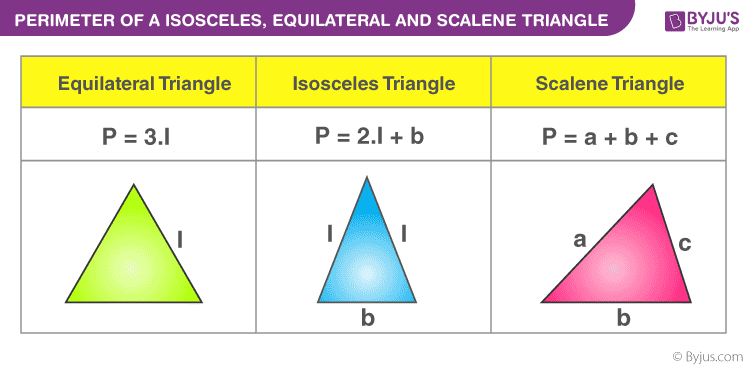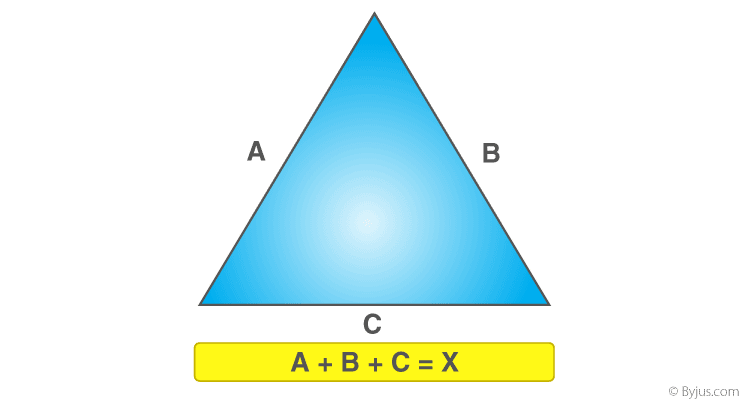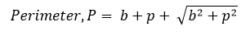# Perimeter of Triangle

Perimeter of Triangle: The perimeter of any two-dimensional figure is defined as the distance around the figure. We can calculate the perimeter of any closed shape just adding up the length of each of the sides. In this article, you will first learn about what is the perimeter, how to find the perimeter of different types of triangles when all side lengths are known. Furthermore, the solved examples will help you to get more views on the topic.

### What is the Perimeter of a Triangle?

The sum of the lengths of the sides is the perimeter of any polygon. In the case of a triangle,

Perimeter = Sum of the three sides

Always include units in the final answer. If the sides of the triangle are measured in centimetres, then the final answer should also be in centimetres.

### Perimeter of Triangle Formula

The formula for the perimeter of a closed shape figure is usually equal to the length of the outer line of the figure. Therefore, in the case of a triangle, the perimeter will be the sum of all the three sides. If a triangle has three sides a, b and c, then,

Perimeter, P = a + b +c

## Perimeter of an Isosceles, Equilateral and Scalene Triangle

Below table helps us to understand how to find the perimeter of different triangles- Equilateral triangle, Isosceles triangle and Scalene triangle.Where a, b, c and l are the side lengths and P = Perimeter.

This formula implies to find the perimeter of a triangle, add the lengths of all of its 3 sides together. If A, B and C are the side measures, and X is perimeter then### Perimeter of Right Triangle

A right triangle has a base(b), hypotenuse(h) and perpendicular(p) as its sides, By the Pythagoras theorem, we know,

h2 = b2 + p2

Therefore, the Perimeter of a right angle triangle= b + p + h## Examples

Let us consider some of the examples on the perimeter of a triangle:

Example 1: Find the perimeter of a polygon whose sides are 5 cm, 4 cm and 2 cm.

Solution: Let,

a = 5 cm

b = 4 cm

c = 2 cm

Perimeter = Sum of all sides = a + b + c = 5 + 4 + 2 = 11

Therefore, the answer is 11 cm.

Example 2: Find the perimeter of a triangle whose each side is 10 cm.

Solution: Since all three sides are equal in length, the triangle is an equilateral triangle.

i.e. a = b = c = 10 cm

Perimeter = a + b + c

= 10 + 10 + 10

= 30

Perimeter = 30 cm.

Example 3: What is the missing side length of a triangle whose perimeter is 40 cm and two sides are 10 cm each?

Solution: Given,

Perimeter = 40 cm

Length of two sides is the same i.e. 10 cm.

Thus, the triangle is an isosceles triangle.

Using formula: P = 2l + b

40 = 2 * 10 + b

40 = 20 + b

or b = 20

Missing side length is 20 cm.

### What does the Perimeter of a Triangle Mean?

The perimeter of a triangle is the total distance around the edges of a triangle. In other words, the length of the boundary of a triangle is its perimeter.

### How to Calculate the Perimeter of a Triangle?

To calculate the perimeter of a triangle, add the length of its sides. For example, if a triangle has sides a, b, and c, then the perimeter of that triangle will be P = a + b + c.

### Calculate the Perimeter of a Right Triangle with Base as 3 cm and height as 4 cm.

First, using the Pythagorean theorem, calculate the hypotenuse of the right triangle.

h =√(base2+perpendicular2)

h = √(32+42)

h = √(9 + 16)

h = √25

Or, h = 5 cm

So, the perimeter of the triangle = 3 + 4 + 5 = 12 cm.

Test your Knowledge on Perimeter of Triangle